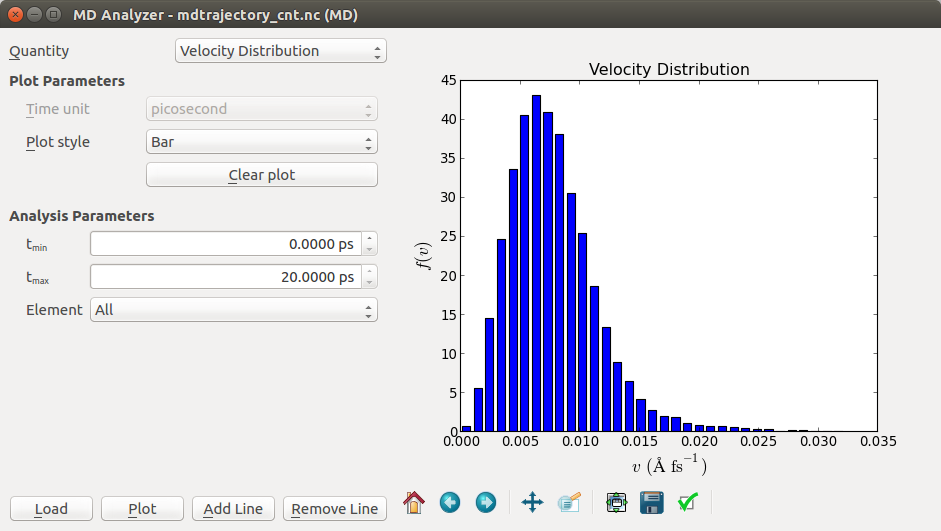# Young’s modulus of a CNT with a defect¶

Carbon nanotubes are well known for their exceptional mechanical properties; Young’s moduli in the 1 TPa range have been measured [KDE+98], which is higher than any other known material. The theoretical Young’s modulus is fairly simple to calculate for a defect-free carbon nanotube, but this is not the case when defects are present. In that case, molecular dynamics (MD) is a suitable tool for calculation of the mechanical properties.

In this tutorial you will build a carbon nanotube (CNT) with a Stone–Wales defect, and use the ATK-ForceField engine to calculate the Young’s modulus.

Important

This tutorial uses a CNT structure that is built in the tutorial Stone–Wales Defects in Nanotubes. If you have not already done so, please go that tutorial first and build the CNT device configuration, or simply download it as a Python script: stone_wales_cnt.py.

## CNT bulk configuration¶

Open the QuantumATK Builder, and use File ‣ Add ‣ From Files to import the previously saved CNT configuration to the Stash. Note that the structure is a device configuration with the Stone–Wales defect in the middle of the central region.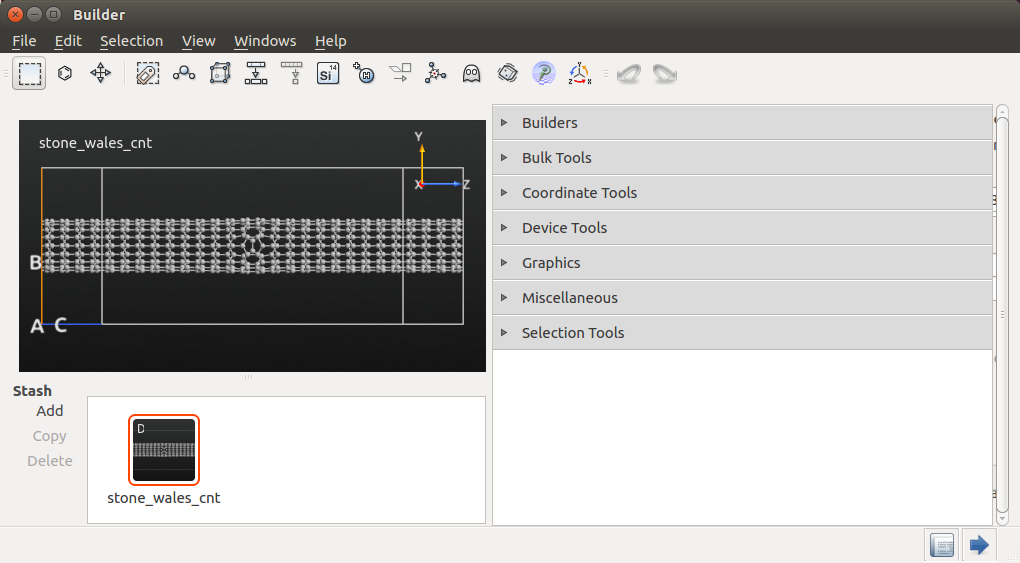Next, highlight the device Stash item, and click the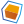icon to convert it to a bulk configuration (the device electrodes are simply removed), and use the Selection Tools ‣ Tags tool to add tags to the atoms at both ends of the nanotube:

• Use the mouse to highlight the left-most carbon atoms and set the tag “Left” on them.
• Do the same for the right-most carbon atoms, as illustrated below.

These tags will be used to impose constraints on the structure during the MD simulation.## Configuring the MD simulation¶

Send the CNT bulk configuration with the Stone–Wales defect to the Script Generator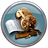and add theNew Calculator and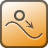Optimization ‣ MolecularDynamics blocks. Then edit both blocks to configure the calculation:

• First, change the default output file to mdtrajectory_cnt.hdf5.
• In the New Calculation block, select the ATK-ForceField calculator, and make sure to use the Tersoff_C_2010 classical potential, which is designed for simulating lattice dynamics of graphene and nanotubes.

Note

For QuantumATK-versions older than 2017, the ATK-ForceField calculator can be found under the name ATK-Classical.

• Make the following changes to the MolecularDynamics block:
• Molecular Dynamics options:
• Set the “Type” to NVT Berendsen.
• Set “Steps” to 20000.
• Initial Velocity options:
• Set “Type” to Maxwell-Boltzmann.
• Set “Temperature” to 300 K.
• Untick the Remove center-of-mass momentum option.
• NVT Berendsen options:
• Set the “Thermal coupling” to 1 fs.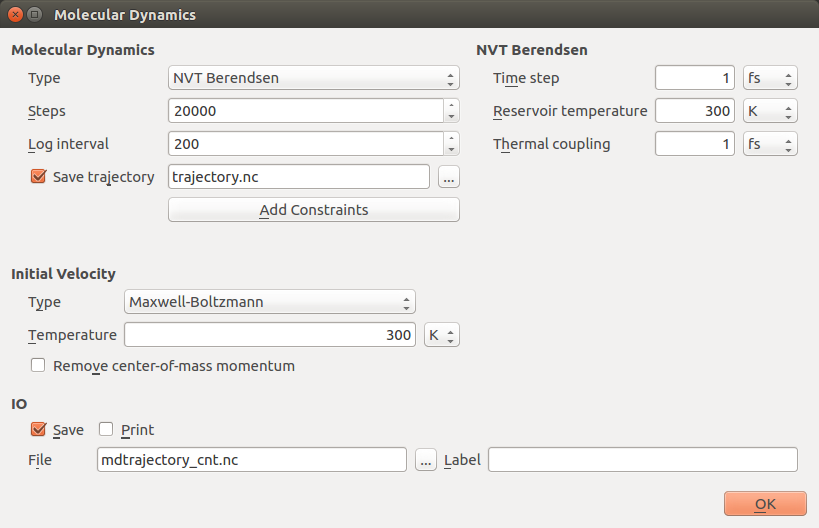Leave the remaining settings at defaults, like in the image above.

Save the script as mdtrajectory_cnt.py and send it to the Editor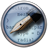by using the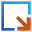icon.

It is possible in QuantumATK to define “hooks”; Python functions or classes that are called before or after each MD step. These hooks can be used to provide customized on-the-fly analysis or monitoring of the system, or – as in this case – to modify the structure dynamically during the simulation.

You will now define a pre-step hook that will be used to strain the nanotube slightly for each step of the MD run:

• With the Python script open in the Editor, scroll down to the bottom of the script and locate the block defining the md_trajectory. The predefined tags “Left” and “Right” can now be used to apply constraints to the system. Change the line

constraints=[]


to

constraints=bulk_configuration.indicesFromTags(["Left","Right"])

• In the md_trajectory block, add the lines

pre_step_hook=strainer,
write_stresses=True,


and add a comma to the line immediately above it (method=method,). The first line will invoke the strainer hook function (defined below), and the second line will ensure that stresses are calculated and stored in the trajectory.

• You should also define what “strainer” is: Add the following line immediately before the md_trajectory block:

strainer = StressStrainUtility()

• The bottom of the script should now look like this:

  1 2 3 4 5 6 7 8 9 10 11 12 13 14 15 16 17 18 19 20 21 22 23 24 25 26 27 28 # ------------------------------------------------------------- # Molecular Dynamics # ------------------------------------------------------------- initial_velocity = MaxwellBoltzmannDistribution( temperature=300.0*Kelvin, remove_center_of_mass_momentum=False ) method = NVTBerendsen( time_step=1*femtoSecond, reservoir_temperature=300*Kelvin, thermostat_timescale=100*femtoSecond, initial_velocity=initial_velocity ) strainer = StressStrainUtility() md_trajectory = MolecularDynamics( bulk_configuration, constraints=bulk_configuration.indicesFromTags(["Left","Right"]), trajectory_filename='mdtrajectory_cnt.hdf5', steps=20000, log_interval=200, method=method, pre_step_hook=strainer, write_stresses=True ) 
• Line 17 above initializes an instance of the class StressStrainUtility, which is a Python class that you now will define. Insert the following at the very top of the script:

  1 2 3 4 5 6 7 8 9 10 11 12 13 14 15 16 17 18 19 20 21 22 23 24 25 26 27 28 29 30 31 32 33 34 35 36 37 38 39 40 41 42 43 44 45 46 47 # Define a prestep function which can expand the system class StressStrainUtility: """ Perform a strain of a configuration over time. """ def __init__(self, delta=0.0001, skip=0): """ @param delta The strain to apply in each step @param first steps to "skip" strain """ self.__delta = [0.0,0.0,delta]*Angstrom self.__skip = skip self.stresses = [] self.lengths = [] def __call__(self, step, time, configuration, forces, stress): """ Strainer call. """ # Get the lattice vectors. u1, u2, u3 = configuration.primitiveVectors() # Don't apply strain in the earliest MD steps if step < self.__skip: return # Store the history self.stresses.append( stress[2,2].inUnitsOf(eV/Angstrom**3) ) self.lengths.append( u3.inUnitsOf(Angstrom) ) # Increase the lattice vectors. u3 = u3 + self.__delta # Set the new lattice. configuration.setBravaisLattice(UnitCell(u1,u2,u3)) # Move all the atoms that are constrained on the right side. constraints = configuration.indicesFromTags("Right") for c in constraints: xyz = numpy.array(configuration.cartesianCoordinates()[c]) + numpy.array(self.__delta) configuration.setCartesianCoordinates(indices=[c], cartesian_coordinates=[xyz]*Angstrom) def length(self): return numpy.array(self.lengths)*Angstrom def stress(self): return numpy.array(self.stresses)*eV/Angstrom**3 

This class defines a method to enable straining of a unit cell along the z-direction. You will use it to study how a CNT with a defect responds to strain.

As you can see from the script, the class has two inputs, delta and skip:

delta
defines how much the u3 vector (the z-direction) is expanded on every step in the MD calculation.
skip
defines how many steps should pass before the configuration will be strained.

The actual straining is done in the “for-loop” beginning at line 38, by increasing the coordinates of the atoms tagged “Right” by the amount delta along the z-direction. On lines 27 and 28, the stress and total length of the configuration are calculated and appended to two separate lists. These will be used later for plotting.

Tip

By writing pre_strain_hook=strainer in the md_trajectory block, the class (technically, the method __call__) is called before the force and energy calculation in every MD step (for more details about hook functions see MolecularDynamics()).

## Computing Young’s modulus¶

To properly calculate the Young’s modulus, it is necessary to take the total volume of the simulation domain into account. The stress reported by QuantumATK therefore has to be multiplied by the total volume of the cell and then divided by the volume of the nanotube.

Several definitions of the volume of a CNT. A common way of defining it is to say that the wall of the nanotube extends for half a $$\pi$$-bond in and out of the wall, so that it becomes a cylindrical shell with thickness equal to that of a $$\pi$$-bond, approximately 1.35 Å, so that the total cross-sectional area is 1.35 Å times the circumference of the nanotube. The circumference for a (5,5)-armchair nanotube is $$5 \times (3 \times x)$$, where $$x$$=1.42 Å is the carbon-carbon bond length. Finally, for the volume, you need the length of the tube, which is given in the .hdf5 file saved by QuantumATK.

• At the bottom of the script, delete the line nlsave('mdtrajectory_cnt.hdf5', md_trajectory).

• Add instead the following lines of code in order to invoke the proper stress calculation:

# -------------------------------------------------------------
# Finding Young's Modulus
# -------------------------------------------------------------

# Find center of cnt from mean x- and y-coordinates
coords = bulk_configuration.cartesianCoordinates()
x_coords = coords[:,0]
y_coords = coords[:,1]
x_0 = numpy.sum(x_coords)/len(x_coords)
y_0 = numpy.sum(y_coords)/len(y_coords)

# Find radius by mean distance from center to atoms' x- and y-coordinates
radius = numpy.sum( ( (x_coords - x_0)**2 + (y_coords - y_0)**2 )**(1/2.0) ) / len(x_coords)

# Find cross-sectional area by multiplying circumference with length of a pi-bond
pi_bond = 1.35
circumference = radius * 2 * numpy.pi
area = circumference * pi_bond

# Find volumes of CNT and of whole cell
volume_cnt = area * strainer.lengths[-1]

u1, u2, u3 = bulk_configuration.bravaisLattice().primitiveVectors()
volume_cell = u1 * u2 * u3

# Factor the stresses to the volume of the CNT
stresses_factored = numpy.array(strainer.stresses * (volume_cell / volume_cnt) )*eV/Angstrom**3

# Calculate the strain
strain = (strainer.length() - strainer.length() ) / strainer.length()

# Calculate Young's modulus in TPa
modulus = (stresses_factored[-1].inUnitsOf(Pa) - stresses_factored.inUnitsOf(Pa) ) / float(strain[-1]) / 1e12
print("Young's Modulus: {} TPa".format(modulus))

• Finally, add a few lines of code that will save different parts of the calculation and plot the results using pylab.

# Save the result to a file.
print(strainer.length())
nlsave("mdtrajectory_cnt.hdf5", md_trajectory, "MD")
nlsave("mdtrajectory_cnt.hdf5",strainer.length(), "C length")
nlsave("mdtrajectory_cnt.hdf5",strainer.stress(), "C stress")
nlsave("mdtrajectory_cnt.hdf5",stresses_factored, "C stresses factored")

# Plot stress-strain curve.
import pylab
pylab.figure()
pylab.plot(strain * 100, stresses_factored.inUnitsOf(GPa))
pylab.title('CNT with Stone-Wales defect')
pylab.xlabel('Strain (%)')
pylab.ylabel('Stress (GPa)')
pylab.grid(True)
pylab.savefig("mdtrajectory_cnt_fig.png")


The script is now ready to be executed. You may download the script to make sure you did the manual editing correctly: mdtrajectory_cnt.py.

Send the script to the Job Manager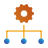by clicking theicon, then save it, and run it. The job should take around 3 minutes on a laptop.

## Visualize and analyse the results¶

The nanotube lengths and stresses that occur during the MD simulation run are saved in the .hdf5 file with different ID’s (“C length”, “C stress”, and “C stresses factored”). These are used to produce the plot titled mdtrajectory_cnt_fig.png, which is automatically saved in the project folder. It shows the stress vs. strain relationship for the nanotube, which is also shown below. The linear (elastic) behavior for small strains make it possible to calculate Young’s modulus E for the nanotube, defined as E = stress/strain.

With this procedure, the calculated Young’s modulus of the carbon nanotube with one Stone–Wales defect is around 2.3 TPa (it may vary across different MD runs). This is pretty far from the calculated value of 1.28 TPa for defect-free nanotubes [SPAS+99]. However, as shown in [KDE+98], nanotubes with defects have in general a higher Young’s modulus.

Note

The figure above shows that the stress is negative for small stress levels, which means that the nanotube is actually under compressive stress until around 1.1% strain.

This could be avoided by running a geometry optimization step before running the MD calculation, which can be done using the Optimization block available in the Script Generator. However, for the strains investigated here, the CNT shows a linear stress–strain behavior, so the obtained Young’s modulus should not change significantly if a geometry optimization is performed first.

The data file mdtrajectory_cnt.hdf5 should be shown on the LabFloor. Select the file and click the Movie Tool in the right-hand plugins panel. The Movie Tool can be used to inspect MD trajectories; it will show a movie of the simulation and data about energies and temperatures. The tool looks like illustrated below.Tip

Right click the mouse and select Show bonds to visualize the bond connection between the carbon atoms.

Another useful plugin is the MD Analyzer, which can show the radial distribution, velocity distribution, and the kinetic energy distribution of the atoms in the system. A screenshot of the velocity distribution is shown below.